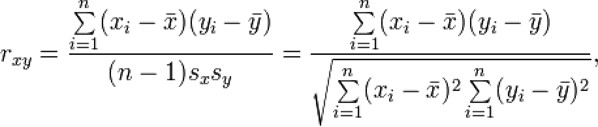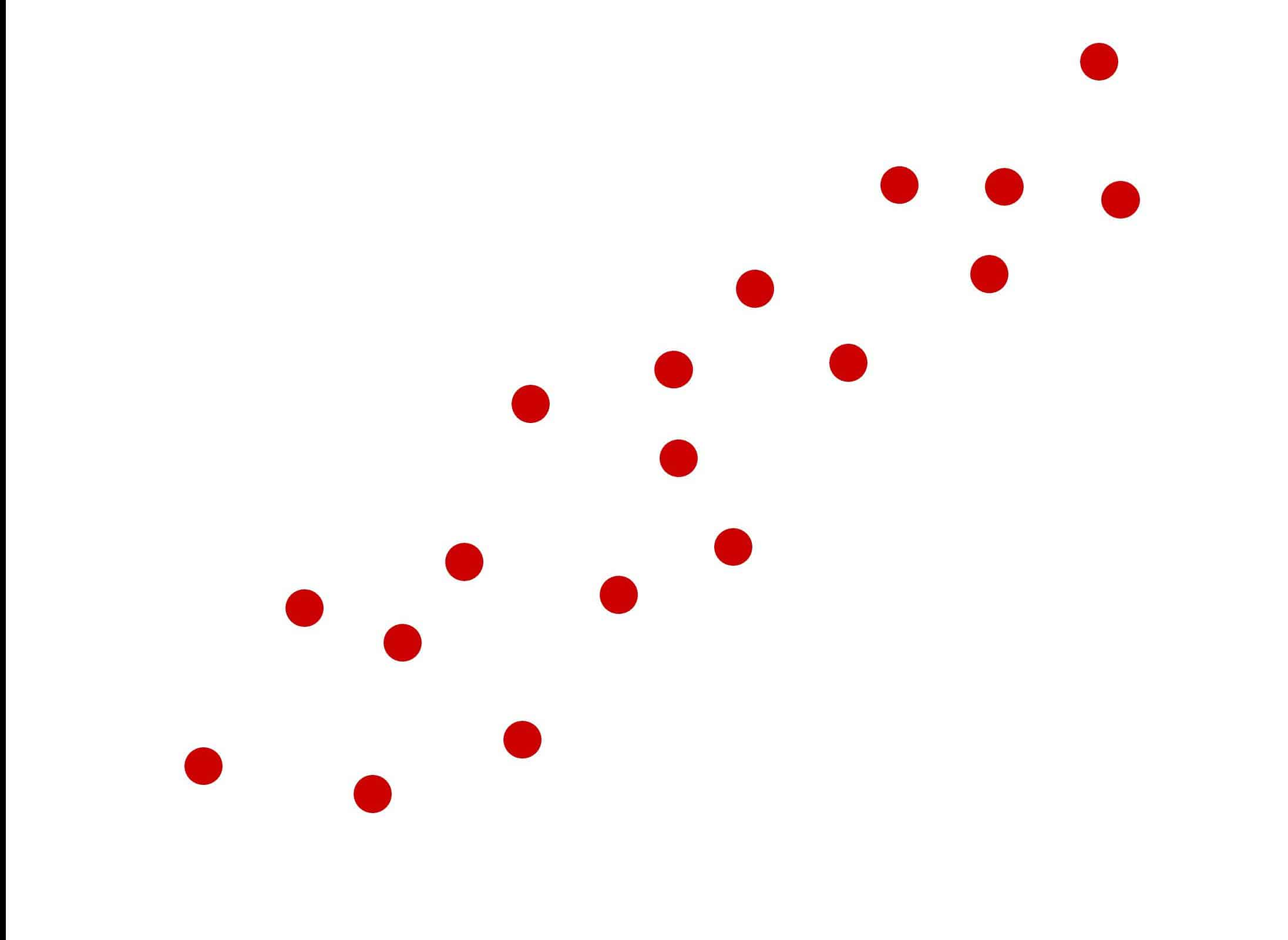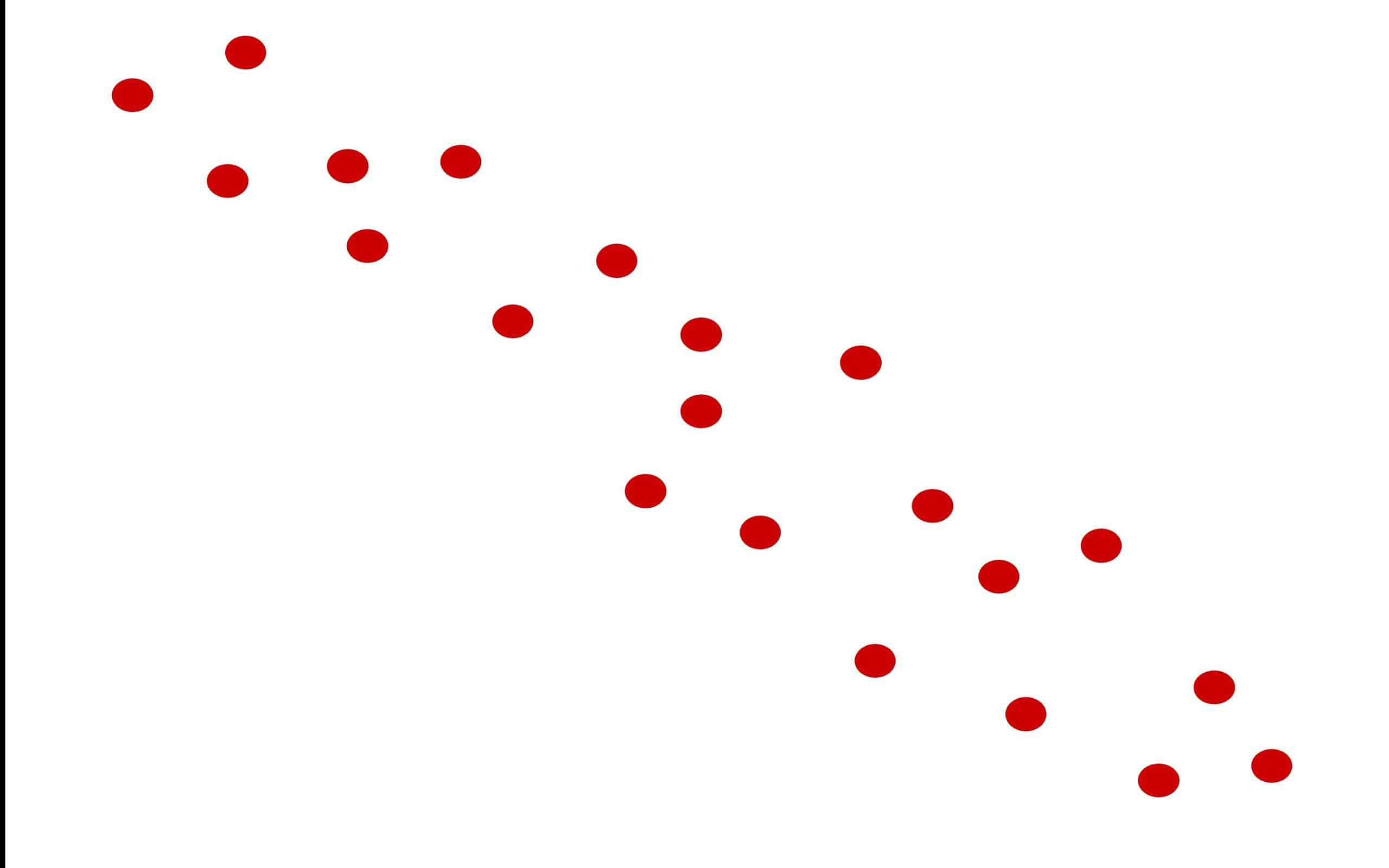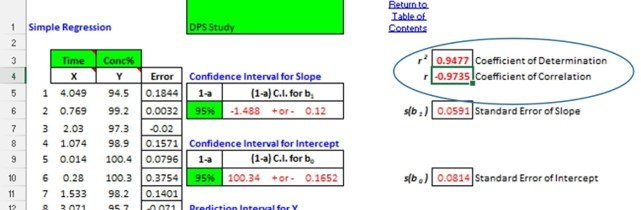You are convinced there is a correlation between your X and Y variables. But, you won’t know for sure until you can establish the strength of the correlation. That’s where R comes in. Let’s learn some more about R.

R is the symbol used to represent the strength and direction of correlation between continuous variables. It is used most often in regression analysis. It is also referred to as the Pearson Correlation Coefficient. It serves as the foundation for the calculation of R Square which is also called the Coefficient of Determination.

## Overview: What is R?

R is a relative measure of the strength of relationship between an independent and dependent variable. The value of R will range between -1 and +1. The -1 value means there is a perfect negative or inverse correlation between your X and Y. An R value of +1 means there is a perfect positive relationship. A value of 0 means there is no correlation. The closer the value of R is to either -1 or +1 the stronger the correlation.

The formula for computing R is:These relationships can best be seen on a scatter plot. Below is a graph showing a moderately strong positive correlation and one showing a moderately inverse correlation.## An industry example of R

The Six Sigma Black Belt (BB) was doing a study for her company of the relationship between time and % concentration of the liquid product. She ran a simple linear regression to understand the relationship. Below is the output from a statistical software package. Note that the R and R square are high numbers close to 1 with the R being close to -1 indicating an inverse relationship.### What is the difference between R and R Square?

R, called the Pearson Correlation Coefficient, measures the direction and relative strength of the relationship between your X and Y variables. R square, also known as the Coefficient of Determination, measures the percent variation in your Y, attributable to the variation in your X or the regression model.

### Can the value of R be negative?

Yes. A negative value for R indicates that the correlation is an inverse relationship. In other words, as your X value increases, your Y value decreases.

### What do the values of R indicate?

The values for R can range from -1 to +1. The closer to either -1 or +1, the stronger the relationship or correlation between your X and Y variables.